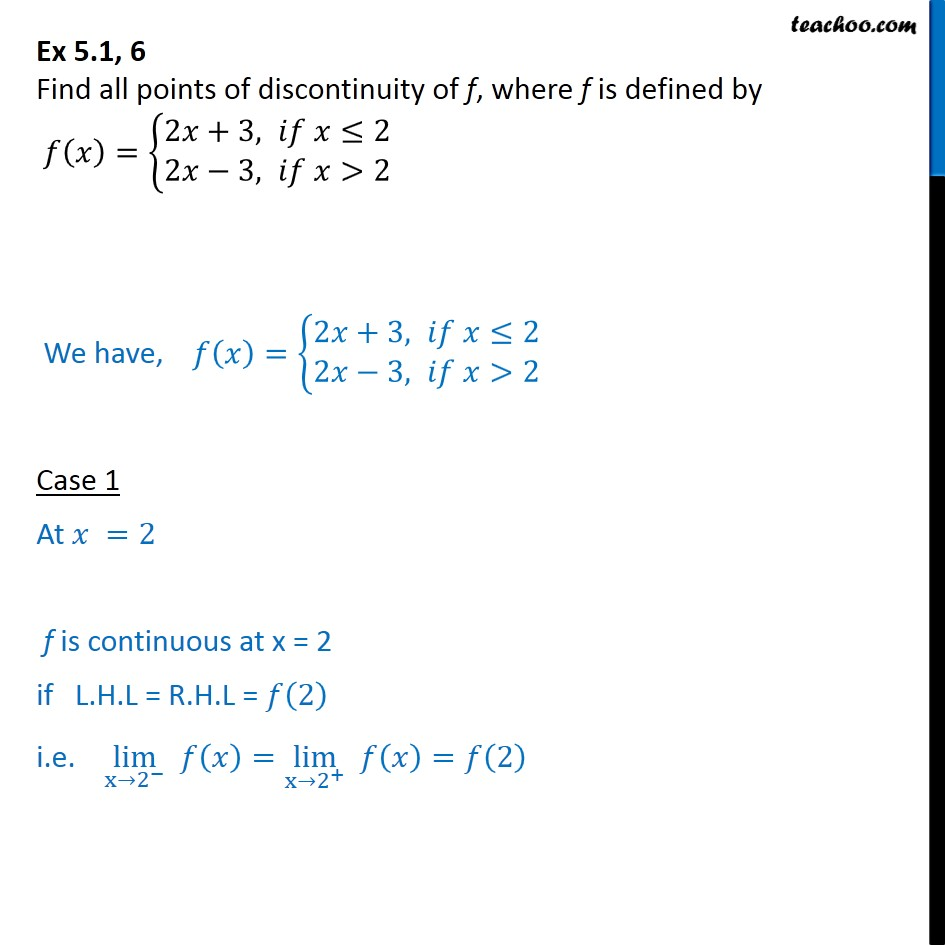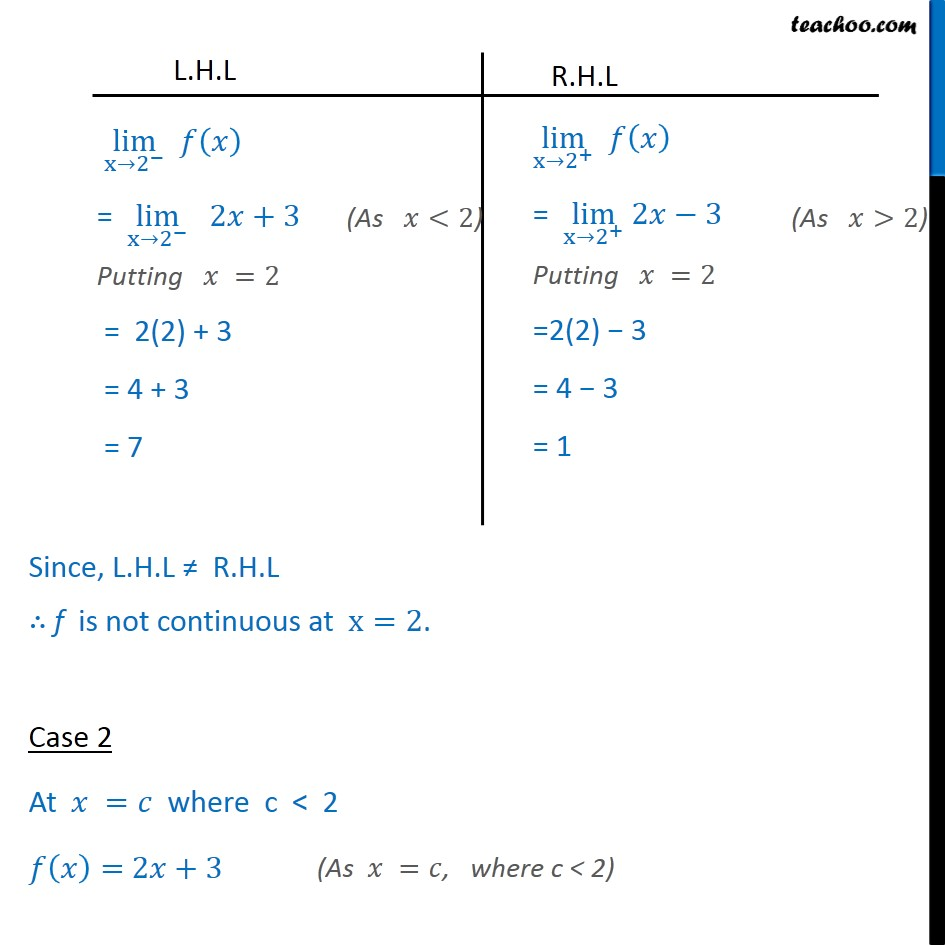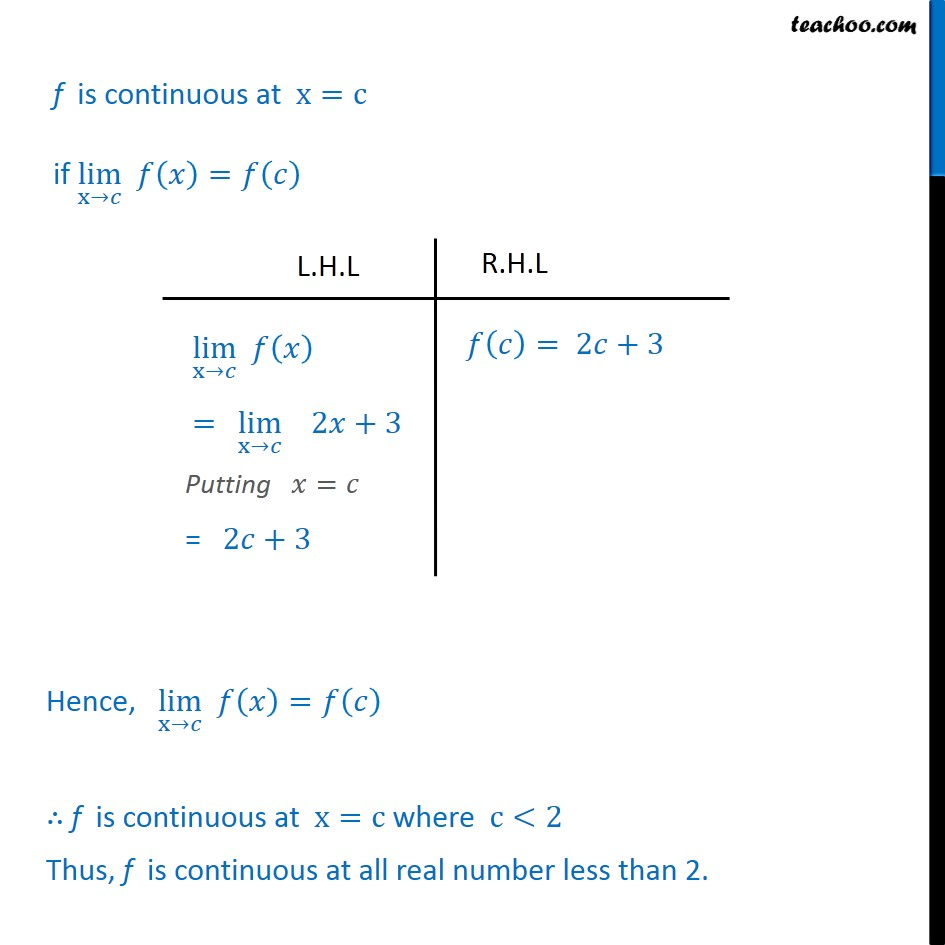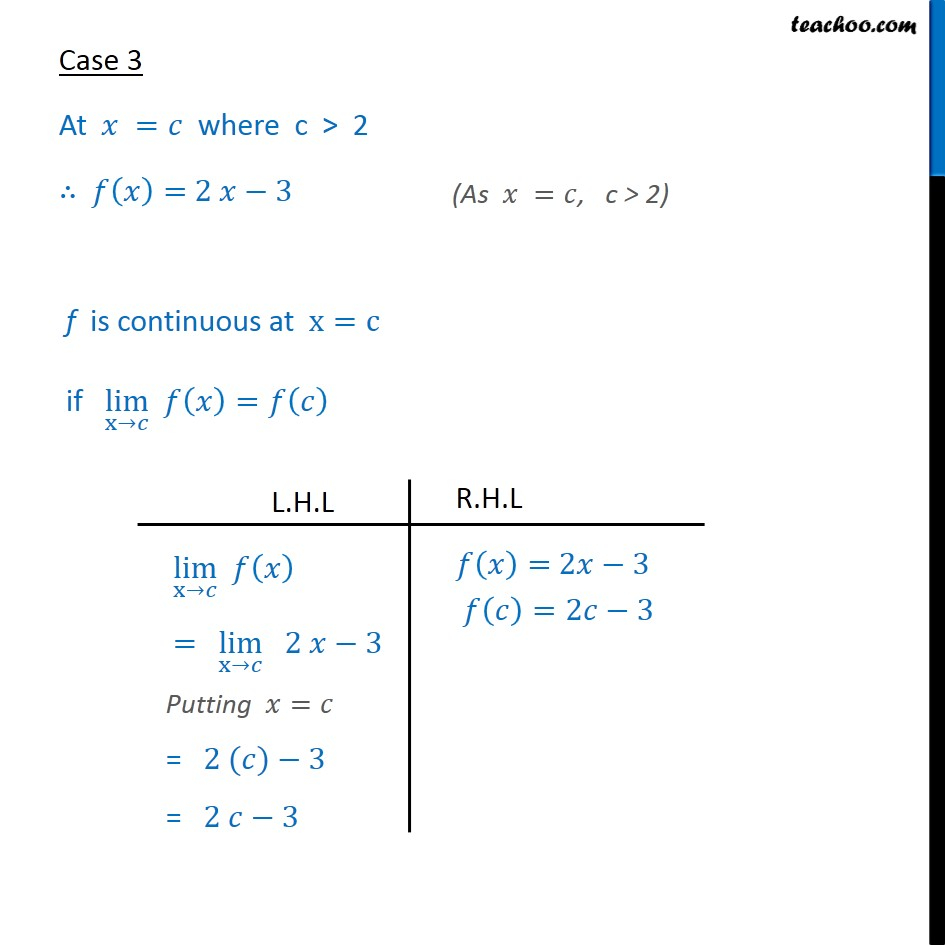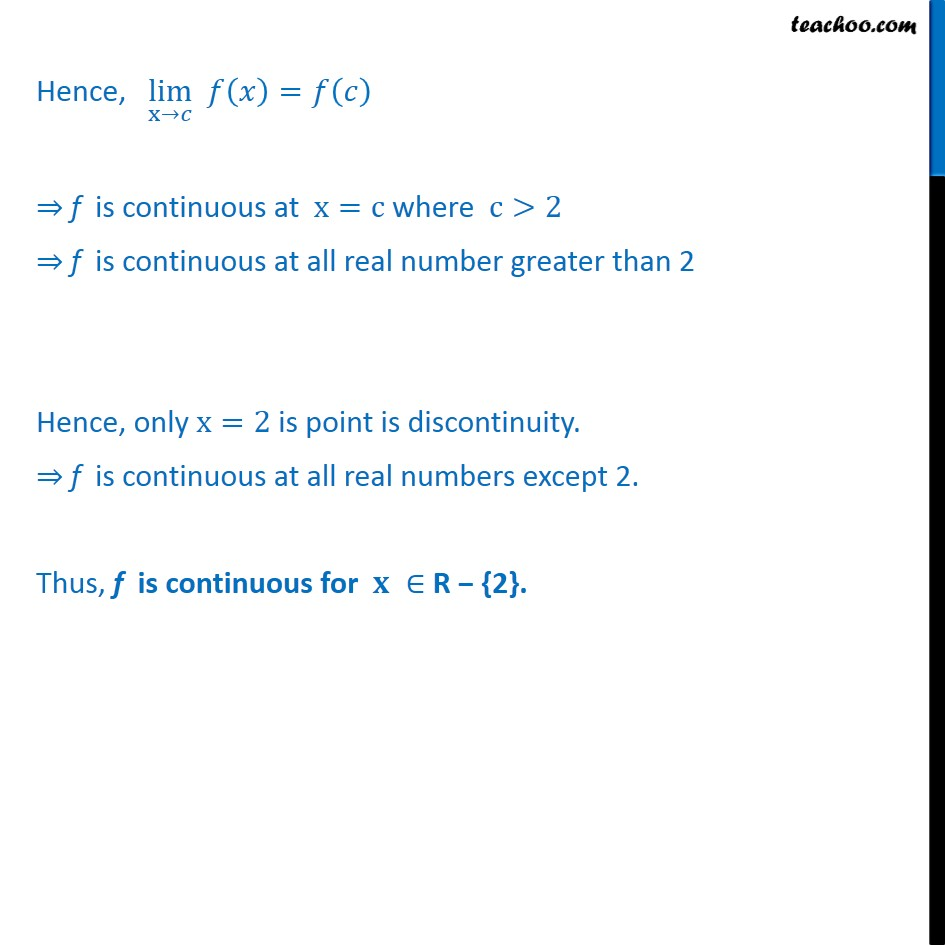1. Chapter 5 Class 12 Continuity and Differentiability
2. Concept wise
3. Checking continuity using LHL and RHL

Transcript

Ex 5.1, 6 Find all points of discontinuity of f, where f is defined by 𝑓(𝑥)={█(2𝑥+3, 𝑖𝑓 𝑥≤2@&2𝑥−3, 𝑖𝑓 𝑥>2)┤ We have, 𝑓(𝑥)={█(2𝑥+3, 𝑖𝑓 𝑥≤2@&2𝑥−3, 𝑖𝑓 𝑥>2)┤ Case 1 At 𝑥 =2 f is continuous at x = 2 if L.H.L = R.H.L = 𝑓(2) i.e. lim┬(x→2^− ) 𝑓(𝑥)=lim┬(x→2^+ ) 𝑓(𝑥)=𝑓(2) L.H.L lim┬(x→2^− ) 𝑓(𝑥) = lim┬(x→2^− ) 2𝑥+3 Putting 𝑥 =2 = 2(2) + 3 = 4 + 3 = 7 R.H.L lim┬(x→2^+ ) 𝑓(𝑥) = lim┬(x→2^+ ) 2𝑥−3 Putting 𝑥 =2 =2(2) − 3 = 4 − 3 = 1 Since, L.H.L ≠ R.H.L ∴ f is not continuous at x=2. Case 2 At 𝑥 =𝑐 where c < 2 𝑓(𝑥)= 2𝑥+3 (As 𝑥 =𝑐, where c < 2) f is continuous at x=c if lim┬(x→𝑐) 𝑓(𝑥)=𝑓(𝑐) L.H.L lim┬(x→𝑐) 𝑓(𝑥) = lim┬(x→𝑐) 2𝑥+3 Putting 𝑥=𝑐 = 2𝑐+3 R.H.L 𝑓(𝑐)= 2𝑐+3 Hence, lim┬(x→𝑐) 𝑓(𝑥)=𝑓(𝑐) ∴ f is continuous at x=c where c<2 Thus, f is continuous at all real number less than 2. Case 3 At 𝑥 =𝑐 where c > 2 ∴ 𝑓(𝑥)= 2 𝑥−3 (As 𝑥 =𝑐, c > 2) f is continuous at x=c if lim┬(x→𝑐) 𝑓(𝑥)=𝑓(𝑐) L.H.L lim┬(x→𝑐) 𝑓(𝑥) = lim┬(x→𝑐) 2 𝑥−3 Putting 𝑥=𝑐 = 2 (𝑐)−3 = 2 𝑐−3 R.H.L 𝑓(𝑥)= 2𝑥−3 𝑓(𝑐)=2𝑐−3 Hence, lim┬(x→𝑐) 𝑓(𝑥)=𝑓(𝑐) ⇒ f is continuous at x=c where c>2 ⇒ f is continuous at all real number greater than 2 Hence, only x=2 is point is discontinuity. ⇒ f is continuous at all real numbers except 2. Thus, f is continuous for 𝐱 ∈ R − {2}.

Checking continuity using LHL and RHL